Also found in: Dictionary, Thesaurus, Medical, Wikipedia.

radioactivity, spontaneous disintegration or decay of the nucleus of an atom by emission of particles, usually accompanied by electromagnetic radiation. The energy produced by radioactivity has important military and industrial applications. However, the rays emitted by radioactive substances can cause radiation sickness, and such substances must therefore be handled with extreme care (see radioactive waste).

Natural radioactivity is exhibited by several elements, including radium, uranium, and other members of the actinide series, and by some isotopes of lighter elements, such as carbon-14, used in radioactive dating. Radioactivity may also be induced, or created artificially, by bombarding the nuclei of normally stable elements in a particle accelerator. Essentially there is no difference between these two manifestations of radioactivity.

The radiation produced during radioactivity is predominantly of three types, designated as alpha (α), beta (β), and gamma (γ) rays. These types differ in velocity, in the way in which they are affected by a magnetic field, and in their ability to penetrate or pass through matter. Other, less common, types of radioactivity are electron capture (capture of one of the orbiting atomic electrons by the unstable nucleus) and positron emission—both forms of beta decay and both resulting in the change of a proton to a neutron within the nucleus—an internal conversion, in which an excited nucleus transfers energy directly to one of the atom's orbiting electrons and ejects it from the atom.

Alpha rays have the least penetrating power, move at a slower velocity than the other types, and are deflected slightly by a magnetic field in a direction that indicates a positive charge. Alpha rays are nuclei of ordinary helium atoms (see alpha particle). Alpha decay reduces the atomic weight, or mass number, of a nucleus, while beta and gamma decay leave the mass number unchanged. Thus, the net effect of alpha radioactivity is to produce nuclei lighter than those of the original radioactive substance. For example, in the disintegration, or decay, of uranium-238 by the emission of alpha particles, radioactive thorium (formerly called ionium) is produced. The alpha decay reduces the atomic number of the nucleus by 2 and the mass number by 4:

Beta rays are more penetrating than alpha rays, move at a very high speed, and are deflected considerably by a magnetic field in a direction that indicates a negative charge; analysis shows that beta rays are high-speed electrons (see beta particle; electron). In beta decay a neutron within the nucleus changes to a proton, in the process emitting an electron and an antineutrino (the antiparticle of the neutrino, a neutral particle with a small mass). The electron is immediately ejected from the nucleus, and the net result is an increase of 1 in the atomic number of the nucleus but no change in the mass number. The thorium-234 produced above experiences two successive beta decays:

Gamma rays have very great penetrating power and are not affected at all by a magnetic field. They move at the speed of light and have a very short wavelength (or high frequency); thus they are a type of electromagnetic radiation (see gamma radiation). Gamma rays result from the transition of nuclei from excited states (higher energy) to their ground state (lowest energy), and their production is analogous to the emission of ordinary light caused by transitions of electrons within the atom (see atom; spectrum). Gamma decay often accompanies alpha or beta decay and affects neither the atomic number nor the mass number of the nucleus.

The nuclei of elements exhibiting radioactivity are unstable and are found to be undergoing continuous disintegration (i.e., gradual breakdown). The disintegration proceeds at a definite rate characteristic of the particular nucleus; that is, each radioactive isotope has a definite lifetime. However, the time of decay of an individual nucleus is unpredictable. The lifetime of a radioactive substance is not affected in any way by any physical or chemical conditions to which the substance may be subjected.

### Half-Life of an Element

The rate of disintegration of a radioactive substance is commonly designated by its half-life, which is the time required for one half of a given quantity of the substance to decay. Depending on the element, a half-life can be as short as a fraction of a second or as long as several billion years.

The product of a radioactive decay may itself be unstable and undergo further decays, by either alpha or beta emission. Thus, a succession of unstable elements may be produced, the series continuing until a nucleus is produced that is stable. Such a series is known as a radioactive disintegration, or decay, series. The original nucleus in a decay series is called the parent nucleus, and the nuclei resulting from successive disintegrations are known as daughter nuclei.

There are four known radioactive decay series, the members of a given series having mass numbers that differ by jumps of 4. The series beginning with uranium-238 and ending with lead-206 is known as the 4n+2 series because all the mass numbers in the series are 2 greater than an integral multiple of 4 (e.g., 238=4×59+2, 206=4×51+2). The accompanying illustration shows a portion of the uranium disintegration series, i.e., from radium-226 to lead-206. The series beginning with thorium-232 is the 4n series, and that beginning with uranium-235 is the 4n+3 series, or actinide series. The 4n+1 series, which begins with neptunium-237, is not found in nature because the half-life of the parent nucleus (about 2 million years) is many times less than the age of the earth, and all naturally occurring samples have already disintegrated. The 4n+1 series is produced artificially in nuclear reactors.

Because the rates of disintegration of the members of a radioactive decay series are constant, the age of rocks and other materials can be determined by measuring the relative abundances of the different members of the series. All of the decay series end in a stable isotope of lead, so that a rock containing mostly lead as compared to heavier elements would be very old.

### Bibliography

A phenomenon resulting from an instability of the atomic nucleus in certain atoms whereby the nucleus experiences a spontaneous but measurably delayed nuclear transition or transformation with the resulting emission of radiation. The discovery of radioactivity by H. Becquerel in 1896 marked the birth of nuclear physics.

All chemical elements may be rendered radioactive by adding or by subtracting (except for hydrogen and helium) neutrons from the nucleus of the stable ones. Studies of the radioactive decays of new isotopes far from the stable ones in nature continue as a major frontier in nuclear research. The availability of this wide variety of radioactive isotopes has stimulated their use in a wide variety of fields, including chemistry, biology, medicine, industry, artifact dating, agriculture, and space exploration. See Alpha particles, Beta particles, Gamma rays, Isotope

A particular radioactive transition may be delayed by less than a microsecond or by more than a billion years, but the existence of a measurable delay or lifetime distinguishes a radioactive nuclear transition from a so-called prompt nuclear transition, such as is involved in the emission of most gamma rays. The delay is expressed quantitatively by the radioactive decay constant, or by the mean life, or by the half-period for each type of radioactive atom, discussed below.

The most commonly found types of radioactivity are alpha, beta negatron, beta positron, electron capture, and isomeric transition. Each is characterized by the particular type of nuclear radiation which is emitted by the transforming parent nucleus. In addition, there are several other decay modes that are observed more rarely in specific regions of the periodic table.

#### Transition rates and decay laws

The rate of radioactive transformation, or the activity, of a source equals the number A of identical radioactive atoms present in the source, multiplied by their characteristic radioactive decay constant λ.

(1)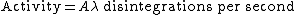Thus Eq. (1) holds, where the decay constant λ has dimensions of s-1. The numerical value of λ expresses the statistical probability of decay of each radioactive atom in a group of identical atoms, per unit time. For example, if λ = 0.01 s-1 for a particular radioactive species, then each atom has a chance of 0.01 (1%) of decaying in 1 s, and a chance of 0.99 (99%) of not decaying in any given 1‒s interval. The constant λ is one of the most important characteristics of each radioactive nuclide: λ is essentially independent of all physical and chemical conditions such as temperature, pressure, concentration, chemical combination, or age of the radioactive atoms.

Many radioactive nuclides have two or more independent and alternative modes of decay. For example, 238U can decay either by alpha-particle emission or by spontaneous fission. When two or more independent modes of decay are possible, the nuclide is said to exhibit dual decay. The competing modes of decay of any nuclide have independent partial decay constants given by the probabilities λ1, λ2, λ3, …, per second, and the total probability of decay is represented by the total decay constant λ, defined by Eq. (2).

(2)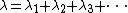The actual life of any particular atom can have any value between zero and infinity. The average or mean life of a large number of identical radioactive atoms is, however, a definite and important quantity. The total L of the life-spans of all the A0 atoms initially present is given by Eq. (3). Then the average lifetime L/A0, which is called the mean life τ, is given by Eq. (4).

(3)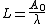(4)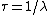The time interval over which the chance of survival of a particular radioactive atom is exactly one-half is called half-period T (also called the half-life, written T1/2). The half-period T is related to the total radioactive decay constant λ, and to the mean life τ, by Eq. (5). For mnemonic

(5)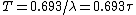reasons, the half-period T is much more frequently employed than the total decay constant λ or the mean life τ.

In a number of cases a radioactive nuclide A decays into a nuclide B which is also radioactive; the nuclide B decays into C which is also radioactive, and so on. For example, 23290Th decays into a series of 10 successive radioactive nuclides. Substantially all the primary products of nuclear fission are negatron beta-particle emitters which decay through a chain or series of two to six successive beta-particle emitters before a stable nuclide is reached as an end product.

#### Alpha-particle decay

Alpha-particle decay is that type of radioactivity in which the parent nucleus expels an alpha particle (a helium nucleus), which contains two protons and two neutrons. Thus, the atomic number, or nuclear charge, of the decay product is 2 units less than that of the parent, and the nuclear mass of the product is 4 atomic mass units less than that of the parent, because the emitted alpha particle carries away this amount of nuclear charge and mass. This decrease of 2 units of atomic number or nuclear charge between parent and product means that the decay product will be a different chemical element, displaced by 2 units to the left in a periodic table of the elements.

In the simplest case of alpha decay, every alpha particle would be emitted with exactly the same velocity and hence the same kinetic energy. However, in most cases there are two or more discrete energy groups called lines. For example, in the alpha decay of a large group of 238U atoms, 77% of the alpha decays will be by emission of alpha particles whose kinetic energy is 4.20 MeV, while 23% will be by emission of 4.15-MeV alpha particles. When the 4.20-MeV alpha particle is emitted, the decay product nucleus is formed in its ground (lowest energy) level. When a 4.15-MeV alpha particle is emitted, the decay product is produced in an excited level, 0.05 MeV above the ground level. This nucleus promptly transforms to its ground level by the emission of a 0.05-MeV gamma ray or alternatively by the emission of the same amount of energy in the form of a conversion electron and the associated spectrum of characteristic x-rays. Thus in all alpha-particle spectra, the alpha particles are emitted in one or more discrete and homogeneous energy groups, and alpha-particle spectra are accompanied by gamma-ray and conversion electron spectra whenever there are two or more alpha-particle groups in the spectrum.

Among all the known alpha-particle emitters, most alpha-particle energy spectra lie in the domain of 4–6 MeV, although a few extend as low as 2 MeV and as high as 10 MeV. There is a systematic relationship between the kinetic energy of the emitted alpha particles and the half-period of the alpha emitter. The highest-energy alpha particles are emitted by short-lived nuclides, and the lowest-energy alpha particles are emitted by the very-long-lived alpha-particle emitters. H. Geiger and J. M. Nuttall showed that there is a linear relationship between log λ and the energy of the alpha particle.

The Geiger-Nuttall rule is inexplicable by classical physics, but emerges clearly from quantum, or wave, mechanics. In 1928 the hypothesis of transmission through nuclear potential barriers was shown to give a satisfactory account of the alpha-decay data, and it has been altered subsequently only in details.

#### Beta-particle decay

Beta-particle decay is a type of radioactivity in which the parent nucleus emits a beta particle. There are two types of beta decay established: in negatron beta decay (β-) the emitted beta particle is a negatively charged electron (negatron); in positron beta decay (β+) the emitted beta particle is a positively charged electron (positron). In beta decay the atomic number shifts by one unit of charge, while the mass number remains unchanged. In contrast to alpha decay, when beta decay takes place between two nuclei which have a definite energy difference, the beta particles from a large number of atoms will have a continuous distribution of energy.

For each beta-particle emitter, there is a definite maximum or upper limit to the energy spectrum of beta particles. This maximum energy, Emax, corresponds to the change in nuclear energy in the beta decay. As in the case of alpha decay, most beta-particle spectra include additional continuous spectra which have less maximum energy and which leave the product nucleus in an excited level from which gamma rays are then emitted.

For nuclei very far from stability, the energies of these excited states populated in beta decay are so large that the excited states may decay by proton, two-proton, neutron, two-neutron, three-neutron, or alpha emission, or spontaneous fission.

The continuous spectrum of beta-particle energies implies the simultaneous emission of a second particle besides the beta particle, in order to conserve energy and angular momentum for each decaying nucleus. This particle is the neutrino. The neutrino has zero charge and extremely small rest mass, travels at nearly the same speed as light (3 × 1010 cm/s), and is emitted as a companion particle with each beta ray. By postulating the simultaneous emission of a beta particle and a neutrino, E. Fermi developed in 1934 a quantum-mechanical theory which satisfactorily gives the shape of beta-particle spectra, and the relative half-periods of beta-particle emitters for allowed beta decays. See Neutrino

When the ground state of a nucleus differing by two units of charge from nucleus A has lower energy than A, then it is theoretically possible for A to emit two beta particles, either β+β+ or β-β- as the case may be, and two neutrinos or antineutrinos, and go from Z to Z ± 2. Here two protons decay into two neutrons, or vice versa. This is a second-order process and so should go much slower than beta decay. There are a number of cases where such decays should occur, but their half-lives are of the order of 1020 years or greater. Such decay processes are obviously very difficult to detect. The first direct evidence for two-neutrino double-beta-minus decay of selenium-82, was found only in 1987.

Whenever it is energetically allowed by the mass difference between neighboring isobars, a nucleus Z may capture one of its own atomic electrons and transform to the isobar of atomic number Z - 1. Usually the electron-capture (EC) transition involves an electron from the K shell of atomic electrons, because these innermost electrons have the greatest probability density of being in or near the nucleus. See Electron capture

#### Gamma-ray decay

Gamma-ray decay involves a transition between two excited levels of a nucleus, or between an excited level and the ground level. A nucleus in its ground level cannot emit any gamma radiation. Therefore gamma-ray decay occurs only as a sequel of another radioactive decay process or of some other process whereby the product nucleus is left in an excited state. Such additional processes include the fusion of two nuclei, Coulomb excitation, and induced nuclear fission. See Coulomb excitation, Nuclear fission, Nuclear fusion

A gamma ray is high-frequency electromagnetic radiation (a photon) in the same family with radio waves, visible light, and x-rays. The energy of a gamma ray is given by hν, where h is Planck's constant and ν is the frequency of oscillation of the wave in hertz. The gamma-ray or photon energy hv lies between 0.05 and 3 MeV for the majority of known nuclear transitions. Higher‒energy gamma rays are seen in neutron capture and some reactions. See Electromagnetic radiation

Gamma rays carry away energy, linear momentum, and angular momentum, and account for changes of angular momentum, parity, and energy between excited levels in a given nucleus. This leads to a set of gamma-ray selection rules for nuclear decay and a classification of gamma-ray transitions as “electric” or as “magnetic” multipole radiation of multipole order 2l, where l = 1 is called dipole radiation, l = 2 is quadrupole radiation, and l = 3 is octupole, l being the vector change in nuclear angular momentum. The most common type of gamma-ray transition in nuclei is the electric quadrupole (E2). There are cases where several hundred gamma rays with different energies are emitted in the decays of atoms of only one isotope. See Multipole radiation

An alternative type of deexcitation which always competes with gamma-ray emission is known as internal conversion. Instead of the emission of a gamma ray, the nuclear excitation energy can be transferred directly to a bound electron of the same atom. Then the nuclear energy difference is converted to energy of an atomic electron, which is ejected from the atom.

When the energy between two states in the same nucleus exceeds 1.022 MeV, twice the rest mass energy of an electron, it is also possible for the nucleus to give up its excess energy to an electron-positron pair—a pair creation process. See Electron-positron pair production

#### Spontaneous fission

This involves the spontaneous breakup of a nucleus into two heavy fragments and neutrons. Spontaneous fission can occur when the sum of the masses of the two heavy fragments and the neutrons is less than the mass of the parent undergoing decay. After the discovery of fission in 1939, it was subsequently discovered that isotopes like 238U had very weak decay branches for spontaneous fission, with branching ratios on the order of 10-6. Some isotopes with relatively long half-lives such as 252Cf have large (3.1%) spontaneous fission branching.

#### Heavy cluster decays

Alpha-particle decay and spontaneous fission are two natural phenomena in which an atomic nucleus spontaneously breaks into two fragments, but the fragments are of very different size in one case and almost equal size in the other. On the basis of fragmentation theory and the two-center shell model, new kinds of radioactivities that are intermediate between alpha-particle decay and fission were predicted in 1980. Subsequently, it was shown theoretically that the new processes should occur throughout a very broad region of the nuclear chart, including elements with atomic numbers higher than 40. However, experimentally observable emission rates could be expected only for nuclei heavier than lead, in a breakup leading to a very stable heavy fragment with proton and neutron numbers equal or very close to Z = 82, N = 126 (20882Pb or its neighborhood). The main competitor is always alpha-particle decay. In 1984, a series of experimental confirmations began with the discovery of 146C radioactivity of 22388Ra. A very promising technique uses solid-state track-recording detectors with special plastic films and glasses that are sensitive to heavier clusters but not to alpha particles.

Proton radioactivity is a mode of radioactive decay that is generally expected to arise in proton-rich nuclei far from the stable isotopes, in which the parent nucleus changes its chemical identity by emission of a proton in a single-step process. Its physical interpretation parallels almost exactly the quantum-mechanical treatment of alpha-particle decay. For many years only a few examples of this decay mode were observed, because of the narrow range of half-lives and decay energies where this mode can compete with other modes. However, in the late 1990s, experimental techniques using new recoil mass spectrometers, which can separate rare reaction products, and new double-sided silicon strip detectors became available and opened up the discovery of many new proton radioactivities. Two-proton radioactivity (the simultaneous emission of two protons) was first observed in 2001 in an excited state of neon-18 and in 2002 in the ground state of iron-45.

#### Delayed particle emissions

Twelve types of beta-delayed particle emissions have been observed. Beta-delayed deuteron (2H) emission, which is not shown there, also can be expected. Over 100 beta-delayed particle radioactivities are now known. Theoretically, the number of isotopes which can undergo beta-delayed particle emission could exceed 1000. Thus, this mode, which was observed in only a few cases prior to 1965, is among the important ones in nuclei very far from the stable ones in nature. Studies of these decays can provide insights into the nucleus which can be gained in no other way.

McGraw-Hill Concise Encyclopedia of Physics. © 2002 by The McGraw-Hill Companies, Inc.
The following article is from The Great Soviet Encyclopedia (1979). It might be outdated or ideologically biased.

the spontaneous transformation of an unstable isotope of a chemical element into another isotope, usually an isotope of another element. The essence of radioactivity lies in the spontaneous change in the composition of the atomic nucleus, which is in either the ground state or a long-lived excited (metastable) state. These transformations are accompanied by the emission of elementary particles or other nuclei, for example, 2He nuclei (alpha particles).

All the known types of radioactive transformations are the result of fundamental microcosmic interactions, that is, strong interactions (nuclear forces) or weak interactions. Strong interactions are responsible for those transformations accompanied by the release of such nuclear particles as alpha particles, protons, and fragments of nuclear fission. Weak interactions are seen in the beta decay of nuclei. Electromagnetic interactions are responsible for quantum transitions, which are accompanied by the emission of gamma radiation, between various energy states of the same nucleus. Since these transitions are not related to a change in the composition of the nucleus, they are not, according to current classification, considered radioactive transformations. The concept of radioactivity has also been extended to include the beta decay of neutrons.

Radioactivity should be distinguished from the transformations of composite nuclei formed during nuclear reactions through the absorption of nuclear particles by target nuclei. The lifetime of such nuclei considerably exceeds the time of flight of the impacting particles (1021—10–22 sec) across distances of the order of nuclear dimensions and may reach 10–13–10–14 sec. Therefore, the lower limit for the lifetime for a radioactive nucleus is arbitrarily taken to be of the order of 10–12 sec.

Types of radioactive transformations. The known types of radioactivity comprise elementary (one-step) transformations and complex (two-step) transformations. Elementary transformations include alpha decay, all types of beta decay (with the release of an electron or positron or with the capture of an orbital electron), spontaneous nuclear fission, proton radioactivity, two-proton radioactivity, and two-neutron radioactivity. In the case of beta decay, the rather long lifetime of the nucleus derives from the nature of the weak interactions. All other types of elementary radioactive processes are caused by nuclear forces. The slowing of such processes to time intervals ≥ 10–12 sec is caused by the presence of potential barriers (coulombic and centrifugal), which hinder the escape of nuclear particles.

Two-step radioactive transformations include processes involving both delayed spontaneous fission and the emission of delayed particles, that is, protons, neutrons, alpha particles, tritium nuclei, and 3He. Delayed processes involve beta decay as a preliminary step, which delays the subsequent, instantaneous emission of nuclear particles. Thus, in the case of two-step processes, the criterion for radioactivity in terms of lifetime is satisfied for the first step only, which occurs as a result of weak interactions.

Historical outline. The discovery of radioactivity dates to 1896, when A. Becquerel found that uranium emits an unknown type of penetrating radiation, which he termed radioactive. Soon afterward, the radioactivity of thorium was discovered, and in 1898, Pierre Curie and his wife, Marie, discovered two new radioactive elements—polonium and radium. The work of these scientists, together with that of E. Rutherford, established the existence and explained the nature of three types of emissions of radioactive elements—alpha rays, beta rays, and gamma rays. In 1903, Rutherford and F. Soddy found that the emission of alpha rays is accompanied by the transmutation of chemical elements, for example, the conversion of radium to radon. In 1913, K. Fajans (Germany) and Soddy independently formulated the displacement laws characterizing the shifting of an isotope in the periodic system of elements under the various radioactive transformations.

In 1934, Frédéric Joliot-Curie and his wife, Irène, discovered artificial radioactivity, which was to acquire great importance. Of the approximately 2,000 presently known radioactive isotopes, only about 300 are natural; the rest are obtained artificially through nuclear reactions. Basically, there is no difference between artificial and natural radioactivity. The study of artificial radioactivity led to the discovery of new types of beta decay—the emission of positrons (I. and F. Joliot-Curie, 1934) and electron capture (L. Alvarez, 1938). The latter had been predicted by H. Yukawa and S. Sakata (Japan, 1935). Subsequently, complex nuclear transformations involving beta decay were discovered. These included the emission of delayed neutrons (J. Dunning and co-workers, USA, 1939), delayed protons (V. A. Karnaukhov and co-workers, USSR, 1962), and delayed nuclear fission (G. N. Flerov and co-workers, 1966–71). The possible emission of delayed 3H and 3He nuclei has been predicted (E. E. Berlovich and Iu. N. Novikov, USSR, 1969). In 1935, I. V. Kurchatov and his co-workers discovered the phenomenon of isomerism, that is, the existence of long-lived excited states in artificially radioactive nuclei.

In 1940, K. A. Petrzhak and Flerov discovered spontaneous nuclear fission. The existence of proton activity had been proposed by Rutherford, and the possibilities of detecting proton radioactivity, as well as the basic characteristics of this fourth type of radioactivity, were considered by B. S. Dzhelepov (1951, USSR). The elementary process of radioactive decay with the emission of protons (from an isomeric state) was first observed experimentally by J. Chernick and his co-workers (USA, 1970). In 1960, V. I. Gol’danskii predicted the existence of two-proton radioactivity, and in 1971, Gol’danskii and L. K. Peker (USSR) predicted two-neutron radioactive nuclear decay (only from an isomeric state).

The law of radioactive decay; units of radioactivity. An exponential decrease in the average number of active nuclei over time is characteristic for processes of radioactive nuclear decay (of nuclei and elementary particles). This law reflects the independence of the decay of a particular nucleus from the decay of the other nuclei. The lifetime of radioactive nuclei is usually characterized by the half-life, that is, the time interval T½ during which the number of radioactive nuclei decreases on the average to one-half the original number. Since the lifetime of an individual nucleus is indeterminate, the exponential law of decay is obeyed only on the average and is the more precise the larger the number of radioactive nuclei involved.

The basic unit of radioactivity is the curie, which was originally defined as the activity of 1 gram of radium. Subsequently, 1 curie was understood as the activity of a radioactive preparation in which there are 3.7 × 1010 disintegrations per second. Fractional units (millicurie, microcurie) and multiple units (ki-locurie, megacurie) are widely used. Another unit of radioactivity is the rutherford, which is equal to 1/3700 curie and corresponds to 106 disintegrations per second.

Alpha decay. Alpha decay is the spontaneous transformation of a nucleus through the ejection of two protons and two neutrons, which form a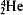nucleus. As a result of alpha decay, the nuclear charge is decreased by 2 units, and the mass number by 4, for example,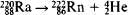The kinetic energy of the emitted alpha particle is determined by the original and final masses of the nucleus and by the mass of the alpha particle. If the final nucleus is formed in an excited state, the kinetic energy is somewhat less; if, on the other hand, an excited nucleus decays, the kinetic energy will be greater. In the latter case, the alpha particles emitted are called long-range alpha particles. The energy spectrum of alpha particles is discrete, and the half-life of alpha-radioactive nuclei is related in an exponential way to the energy of the ejected alpha particles (Geiger-Nuttall rule). The theory of alpha decay, based on a quantum-mechanical description of the penetration of a potential barrier, was developed in 1928 by G. Gamow and, independently, by the British physicist R. Gurney and the American E. Condon.

More than 200 alpha-radioactive nuclei are known. They are located mainly at the end of the periodic table, after Pb, for which the filling of the proton nuclear shell is concluded with Z = 82. About 20 alpha-radioactive isotopes of the rare-earth elemerits are also known. Here, alpha-decay is more characteristic for nuclei with 84 neutrons, which upon the ejection of alpha particles are converted into nuclei with a filled neutron nuclear shell (N = 82). The lifetimes of alpha-radioactive nuclei vary within a wide range, from 3 × 10–7 sec (for 212Po) to 2–5 × 1015 years (for the natural isotopes 142Ce, 144Nd, and 174Hf). The energy of the observed alpha decay lies between 4 and 9 megaelec-tron volts (MeV)—with the exception of long-range alpha particles—for all heavy nuclei and between 2 and 4.5 MeV for the rare-earth elements.

Beta decay. Beta decay (β-decay) is the spontaneous inter-conversion of protons and neutrons. It occurs within the nucleus and is accompanied by the emission or absorption of electrons (e) or positrons (e+) and neutrinos (ve) or antineutrinos (e):

(1) Electron β--decay: npβ- = e- + e. For example,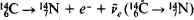.

(2) Positron β+-decay: pnβ+ + e+ + ve. For example,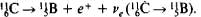(3) Electron capture: p + e-nβ+ + ve. For example,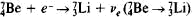.

Electrons are captured from one of the atomic orbits, usually from the K shell, which is closest to the nucleus (K capture), more rarely from the succeeding L and M shells (L and M capture). Electron β--decay is characteristic of neutron-rich nuclei, in which the number of neutrons is greater than the number in stable nuclei (and of nuclei where Z > 83, if the number of neutrons is greater than the number in beta-stable nuclei, which experience only alpha decay). Positron β+-decay and electron capture are characteristic of neutron-deficient nuclei, which are lighter than stable or beta-stable nuclei. The energy in beta decay is distributed among three particles, namely, an electron or positron, an antineutrino or neutrino, and the product nucleus. Thus, the spectrum of beta particles is continuous. Beta-radioactive isotopes are encountered for all elements of the periodic system.

One characteristic of electron capture is the weak dependence of the rate of capture on the chemical state of the atoms undergoing transformation. The nucleus captures an electron from one of the electron shells of the atom, and the probability of capture is determined by the structure not only of the inner shell, which transfers an electron to the nucleus, but also to a lesser extent by the structure of the more distant shells, including the valence shells. The change in the charge of the nucleus in beta decay leads to a subsequent rearrangement (“scrambling”) of the electron shells of the atoms, excitation, ionization of atoms and molecules, and the breaking of chemical bonds. The chemical consequences of beta decay and, to a lesser extent, of other radioactive transformations are the subject of many studies in radiochemistry.

Spontaneous fission. Spontaneous fission is the spontaneous decay of heavy nuclei into two or, less frequently, three or four fragments, which are the nuclei of elements in the middle of the periodic system. Spontaneous fission and alpha decay limit the possibilities of obtaining new transuranium elements.

Proton and two-proton radioactivity. Proton and two-proton radioactivity is the spontaneous decay of neutron-deficient nuclei with the emission of either one or, simultaneously, two protons, which penetrate the coulombic barrier by the tunneling effect. Two-proton radioactivity is made possible by the pairing of protons with opposite spins in the nucleus, a pairing accompanied by the liberation of approximately 2 MeV of energy. As a result of this pairing, the simultaneous emission of a pair of protons from the nucleus requires the expenditure of less energy than the removal of one of these protons from the other. In some cases, the emission of a proton pair is accompanied by the liberation of energy (over a period >10–12 sec), whereas the ejection of a lone proton would require the expenditure of energy.

The difficulties in observing proton and two-proton radioactivity are caused both by the short (compared to other types of radioactivity) lifetimes of the nuclei and by the pronounced neutron deficiency of these nuclei. The nuclear reactions that produce these nuclei are therefore improbable because they are accompanied by the ejection of a large number of neutrons. Proton radioactivity has thus far been observed only in the decay of the nucleus of cobalt, not in the ground state, but an excited (isomeric) state of 53MCo. Two-proton radioactivity, as well as two-neutron decay, has not yet been experimentally detected.

Gamma rays; nuclear isomers. The emission of gamma rays accompanies radioactivity in those cases where the daughter nuclei are formed in excited states. The lifetimes of nuclei in such excited states are determined by the properties (spin, parity, and energy) both of the given level and of lower-lying levels to which transitions may occur with the emission of gamma rays. The duration of gamma transitions increases sharply as the energy of the transitions decreases and as the difference of the angular momenta of the original and final nuclear states increases. In a number of cases, the duration is significantly greater than 10–10–10–9 sec; that is, in addition to the ground state of a stable or radioactive nucleus, a metastable excited (isomeric) state may exist for relatively long periods, sometimes for years. For many nuclear isomers, the phenomenon of internal electron conversion is observed. Here, the excited nucleus does not emit gamma rays and instead transfers its excess energy to the electron shells, which results in the ejection of an electron from the atom. After internal conversion, secondary radiation in the X-ray and optical ranges arises as a result of the filling of the unoccupied site by another electron and the subsequent transitions. The participation of electron shells in conversion transitions means that the lifetime of the corresponding isomers is dependent, though only slightly, on the chemical state of the atoms undergoing transformation.

Isomers are known for which decay through one of the basic types of radioactivity predominates over gamma radiation and the formation of another state of the same isotope. Thus, the isomer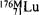(T½ = 3.7 hours) undergoes beta decay, as does the ground-state isotope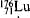; the isomer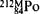(T½ = 45 sec) undergoes alpha decay, as does the ground-state isotope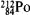; and the isomer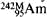(T½ = 14 milliseconds) undergoes spontaneous fission.

Radioactive series (families). In many cases, the products of radioactive decay are themselves radioactive, and the formation of a stable isotope is preceded by a chain of radioactive decay processes. Examples of such chains are the radioactive series of the natural isotopes of heavy elements, which begin with the nuclides 238U, 235U, and 232Th and terminate with such stable isotopes of lead as 206Pb, 207Pb, and 208Pb. Many radioactive isotopes can decay through two or more of the basic types of radioactivity mentioned above. As a result of this competition between various paths of decay, branching of the radioactive transformations will occur. Branching arising from the possibility of either alpha or electron beta decay is characteristic of natural radioactive isotopes. For isotopes of transuranic elements, branching arising from the competition between alpha (less frequently, electron beta) decay and spontaneous fission is more common. Competition between positron beta decay and electron capture is often observed in neutron-deficient nuclei. For many isotopes with odd Z and even A, two opposite variants of beta decay are possible in terms of energy—electron beta decay with electron capture and electron beta decay with positron beta decay.

Conclusion. The discovery of radioactivity has had an enormous effect on the development of science and technology. This discovery marked the beginning of a period of intense study of the properties and structure of matter. New possibilities arising from the application of nuclear energy to power engineering, industry, and many other areas of human activity all stem from the discovery of the capacity of chemical elements to undergo spontaneous transformations. More than ten Nobel prizes in physics and chemistry have been awarded for work related to the study and use of radioactivity. Recipients include A. Becquerel, P. Curie and M. Curie, E. Fermi, E. Rutherford, F. Joliot-Curie and I. Joliot-Curie, G. de Hevesy, O. Hahn, E. McMillan and G. Seaborg, and W. Libby.

### REFERENCES

Davydov, A. S. Teoriia alomnogo iadra. Moscow, 1958.
Haissinsky, M. N. Iadernaia khimiia i ee prilozheniia. Moscow, 1961. (Translated from French.)
Eksperimental’naia iadernaia fizika, vol. 3. Edited by E. Segre. Moscow, 1961. (Translated from English.)
Uchenie o radioaktivnosti: Istoriia i sovremennost’. Moscow, 1973.

V. 1. GOL’DANSKII and E. M. LEIKIN

[‚rād·ē·ō·ak′tiv·əd·ē]
(nuclear physics)
McGraw-Hill Dictionary of Scientific & Technical Terms, 6E, Copyright © 2003 by The McGraw-Hill Companies, Inc.

the spontaneous emission of radiation from atomic nuclei. The radiation can consist of alpha, beta, and gamma radiation
Collins Discovery Encyclopedia, 1st edition © HarperCollins Publishers 2005
References in periodicals archive ?
The soil under these trees was thus less contaminated by radioactivity. The leaves of evergreen trees located higher in the crown were also more contaminated than those located lower.
And despite the low levels of radioactivity detected by monitors, findings suggested that those in close range of its origin would have needed to seek shelter to protect themselves from exposure, NPR reported.
, in which the radioactivity yield of [sup.18]F increases with increasing proton dose.
Radioactivity in drinking water can spike for different reasons, such as a Fukushima-type nuclear power meltdown or a radiopharmaceutical transport spill.
"Much of the work we present here was used to develop and validate a rapid radioactivity measurement method for a federal agency," says lead author Andrew Nelson, a Presidential Graduate Research Fellow and PhD candidate in human toxicology.
In all three locations, the levels of the liquid wouldn't expose radioactivity to a level greater than the annual exposure limit set by the UK Environment Agency.
Wetzel-the other landfill taking large amounts of the waste-also showed radioactivity.
However, the company noted on Friday that the previous radioactivity levels had been wrong, meaning that it was also likely reading taken from the other wells at the disaster-struck plant prior to September were also likely to have been inaccurate, the Asahi Shimbum newspaper reported.